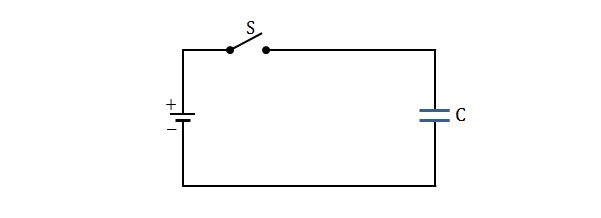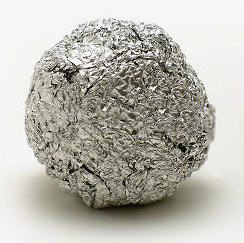Electricity and Magnetism

# CapacitorsThe two metal plates in the above figure have net charges of $$+140.0\text{ pC}$$ and $$-140.0\text{ pC},$$ respectively. If the potential difference between them is $$10.0\text{ V},$$ what is the capacitance of the system consisting of the two metal plates?The capacitor in the above figure has a capacitance of $$40.0 \,\mu\text{F}$$ and is initially uncharged. The battery provides a potential difference of $$90.0\text{ V}.$$ After switch S is closed, how much charge will pass through it?

Consider a spherical capacitor which consists of two concentric spherical shells of radii $$38.0\text{ mm}$$ and $$40.0\text{ mm},$$ respectively. If we want to make a parallel-plate capacitor with the same separation, what must be the area of each plate for the same capacitance?

The value of electrostatic constant is $$\displaystyle k=\frac{1}{4\pi\varepsilon_0}=8.99 \times 10^9 \text{ N}\cdot\text{m}^2\text{/C}^2$$ and the value of the permittivity constant is $$\varepsilon_0=8.85 \times 10^{-12} \text{ C}^2\text{/N}\cdot\text{m}^2.$$One can make a homemade capacitor using an aluminum foil and a plastic or a wooden stick (it's important that the stick is an insulator). Make a small ball out of the aluminum foil and wrap it around the stick. Then make a wider foil sphere around the ball so that they don't touch, and your capacitor is ready!

If the radius of the smaller ball is $$5~\mbox{cm}$$ and of the bigger sphere $$15~\mbox{cm}$$, which capacitance in pF do you expect to obtain?

Details and assumptions

• The ball and the sphere are centered around the same point

If you want to make a parallel-plate capacitor of capacitance $$0.9\text{ pF}$$ with two metal plates with area $$1.1\text{ cm}^2,$$ what must be the separation between the two plates?

The value of the permittivity constant is $$\varepsilon_0=8.85 \times 10^{-12} \text{ C}^2\text{/N}\cdot\text{m}^2.$$

×

Problem Loading...

Note Loading...

Set Loading...